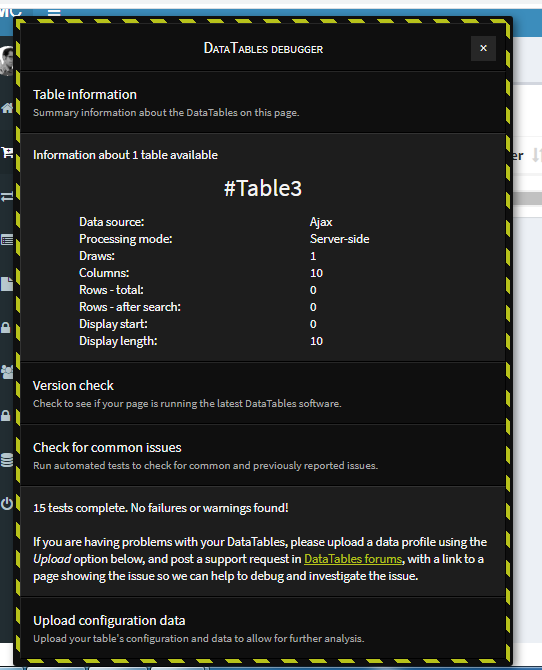# Error memory limit 10240KB exceeded for buffered on getting records from DB to Datatables (sqlsrv)

edited January 14

in other tables is working perfectly except one .. idk why it shows momory limit when i, try to get records from DB to Datatables. is there something wrong in my script ?

My JS Script

``````\$(document).ready(function() {
\$('#Table3').DataTable( {
"processing": true,
"serverSide": true,
"ajax":{
"url": "<?php echo base_url().'Penjualan/get_ajax';?>",
"type": "POST"
}
} );
} );
``````

**My Controller **

`````` function get_ajax() {
\$list = \$this->Penjualan_model->get_datatables();
\$data = array();
\$no = @\$_POST['start'];
foreach (\$list as \$item) {
\$no++;
\$row = array();
\$row[] = \$no;
\$row[] = \$item->no_bukti;
\$row[] = \$item->tgl_jual;
\$row[] = \$item->kode_ctr;
\$row[] = \$item->nama_ctr;
\$row[] = \$item->kode_mcm;
\$row[] = \$item->id_mcm;
\$row[] = \$item->nama_mcm;
\$row[] = '<button type="button" class="detail btn btn-info btn-xs" data-toggle="modal" value="'.\$item->no_bukti.'" id="view"
data-no_bukti="'.\$item->no_bukti.'"
data-tgl_rtr="'.\$item->tgl_jual.'"
data-nama_ctr="'.\$item->nama_ctr.'"
data-nama_alasan="'.\$item->nama_mcm.'">
<i class="fa fa-eye"></i></button>
<button type="button" class="btn btn-danger btn-xs" value="'.\$item->no_bukti.'" id="delete"><i class="fa fa-trash"></i></button>';
\$data[] = \$row;
}
\$output = array(
"draw" => @\$_POST['draw'],
"recordsTotal" => \$this->Penjualan_model->count_all(),
"recordsFiltered" => \$this->Penjualan_model->count_filtered(),
"data" => \$data,
);
// output to json format
echo json_encode(\$output);
}
``````

**My model **

``````  // start datatables
var \$column_order = array('no_bukti', 'tgl_jual', 'kode_ctr', 'nama_ctr', 'a.kode_mcm', 'c.id_mcm', 'c.nama_mcm'); //set column field database for datatable orderable
var \$column_search = array('no_bukti', 'tgl_jual', 'a.kode_ctr', 'nama_ctr', 'a.kode_mcm', 'c.id_mcm', 'c.nama_mcm'); //set column field database for datatable searchable
var \$order = array('no_bukti' => 'asc'); // default order

private function _get_datatables_query() {
\$this->db->select('a.*,b.nama_ctr,c.nama_mcm');
\$this->db->from('mcmjualh a');
\$this->db->join('mcmcenter b', 'b.kode_ctr = a.kode_ctr');
\$this->db->join('mcmmcm c', 'c.kode_mcm = a.kode_mcm');
\$i = 0;
foreach (\$this->column_search as \$item) { // loop column
if(@\$_POST['search']['value']) { // if datatable send POST for search
if(\$i===0) { // first loop
\$this->db->group_start(); // open bracket. query Where with OR clause better with bracket. because maybe can combine with other WHERE with AND.
\$this->db->like(\$item, \$_POST['search']['value']);
} else {
\$this->db->or_like(\$item, \$_POST['search']['value']);
}
if(count(\$this->column_search) - 1 == \$i) //last loop
\$this->db->group_end(); //close bracket
}
\$i++;
}

if(isset(\$_POST['order'])) { // here order processing
\$this->db->order_by(\$this->column_order[\$_POST['order']['0']['column']], \$_POST['order']['0']['dir']);
}  else if(isset(\$this->order)) {
\$order = \$this->order;
\$this->db->order_by(key(\$order), \$order[key(\$order)]);
}
}
function get_datatables() {
\$this->_get_datatables_query();
if(@\$_POST['length'] != -1)
\$this->db->limit(@\$_POST['length'], @\$_POST['start']);
\$query = \$this->db->get();
return \$query->result();
}
function count_filtered() {
\$this->_get_datatables_query();
\$query = \$this->db->get();
return \$query->num_rows();
}
function count_all() {
\$this->db->from('mcmjualh');
return \$this->db->count_all_results();
}
// end datatables
``````

Edited by Colin - Syntax highlighting. Details on how to highlight code using markdown can be found in this guide.

It would be worth adding debug to those scripts - to see if it's getting stuck in a loop somewhere.

Colin

• i didnt found anything from debugger .. but total rows should be 10 just like in other table but in this one is 0 ..